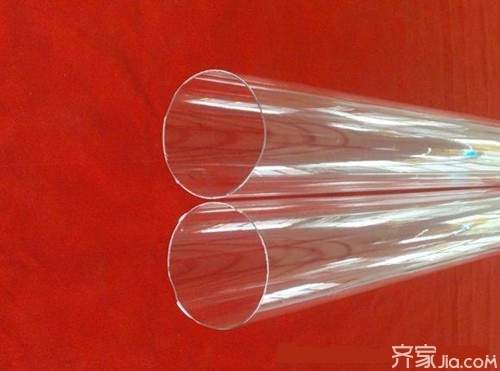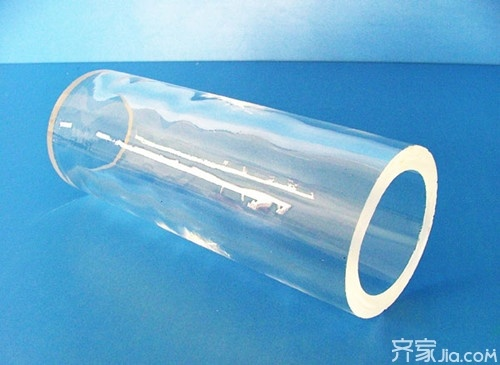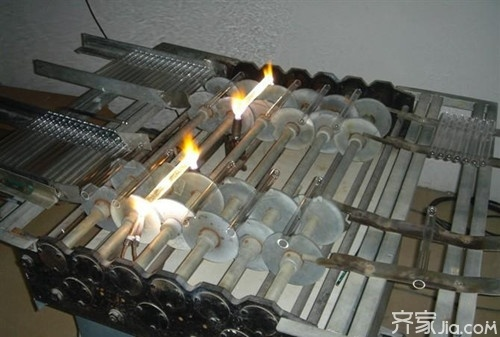|

# 石英管厂家推荐 石英管市场报价及性能

石英玻璃管是用二氧化硅制造的特种工业技术玻璃，是一种非常优良的基础材料。石英玻璃具有一系列优良的物理、化学性能。用于电火桶,电烤火炉,电取暖器里面,起发热作用。现在市面上石英管厂家有很多，产品质量良莠不齐，消费者在选择的时候总是犹豫不决。今天在这里为大家推荐几个不错的石英管厂家，希望对大家的选购有所帮助。石英管加热原理

石英管是采用了经特殊工艺加工的乳白石英玻璃管、配用电阻合材料作为发热子，由于乳白石英玻璃可以吸收来自电热丝辐射的几乎全部的可见光和近红外光、且能使之转化为远红外辐射。但目前的工业用红外线加热管基本淘汰了乳白色石英管，因其料性比较脆，故不能形成很长的乳白色加热管。且乳白色具有遮光效应，阻止了其热量。因其管壁温度高，故而只适用于近红外加热。

石英管技术特征

(1)无需远石英涂层，光谱辐射匹配吸收特性好，长期使用辐射性能不退变，电热转换效率高。比一般加热元件节能30%左右。

(2)工作温度高，选择范围宽。其中用陶瓷帽封装型的，最高耐温达800℃。

(3)升温快、热惯性小，耐高温、耐腐蚀，热化学性能稳定性好，使用寿命长，绝缘强度高。

(4)无污染，是一种清洁型的加热管，符合现代生产高节奏、高品质的要求，适用于卫生要求高的家用电器上。

(5)设计、制造、安装都十分方便，经济安全。石英管化学性能

耐高温·石英管

石英玻璃的软化点温度约1730℃，可在1100℃下长时间使用，短时间最高使用温度可达1450℃，

耐腐蚀·石英管

除氢氟酸外，石英玻璃几乎不与其他酸类物质发生化学反应，其耐酸能力是陶瓷的30倍，不锈钢的150倍，尤其是在高温下的化学稳定性，是其他任何工程材料都无法比拟的。

石英管规格

规挌按你需要而定，规格包括外径，壁厚，长度。各厂有不同产品，所以规格都不一样。因为是电熔炉生产，出耒的产品外径与壁厚不可能完全一样，有一定的出入。

石英管价格

石英管价格上下浮动可能是会比较大，因为石英管的材质也会有好坏之分，口径大、材质更优良的石英管价格自然会更高些。而且这种贵一些的石英管的耐热性等各个方面的性能都会好些，所以一分价格一分货这句话是一点都不错。不过对于购买石英管小编还是建议大家买质量好些的，以为如果你买到一些比较劣质的石英管它的加热性能和耐热性能可能都会受到影响。石英管厂家推荐

1、连云港富彩矿物制品有限公司

连云港富彩矿物制品有限公司位于中国水晶之都东海，这里拥有丰富的石英矿产资源。公司长期生产供应石英玻璃管。产品种类齐全，规格多样。为了适应大尺寸晶圆片及太阳电池片扩散的需要，本公司对直拉法生产的Φ320mm透明石英管进行再次整形，最大可生产出Φ460mm微电子及太阳电池扩散用的炉芯管。由于生产成本降低，产品在市场上独具价格优势，已大量出口韩、美、台湾等国家和地区。

2、连云港创通石英制品有限公司

连云港创通石英制品有限公司是国内知名的石英制品及深加工的专业厂家。公司生产与经营产品有：透明石英玻璃管、滤紫外线石英玻璃管及其他特种用途石英制品等。公司还对石英玻璃管深加工，有切割、烧口、封口、弯曲、磨砂、异形等，工艺技术精湛，在同行业具有较高水平。产品广泛应用于电光源、半导体、电加热、红外加热、光学通讯、化工等领域，深受用户好评。

3、东海县宏伟石英制品有限公司

连云港市东海县宏伟石英制品有限公司位于“中国石英中心”——江苏省东海县。主要从事光伏及研发大规模集成电路生产用的配套材料——高品质石英玻璃制品以及生产电光源、杀菌消毒、化工管道、通讯、加热设备等行业使用的石英玻璃管材。

其中大规模集成电路用直径320大口径高纯石英管是江苏省科技支撑(工业)项目。最新研发的直径560大口径高纯石英管材已经通过了国家级新品预审;该公司新产品14寸以上晶圆氧化(扩散)炉关键部件--大口径高纯石英玻璃管研发及产业化已申请江苏省科技成果转化专项资金;公司产品除畅销国内外二十多个省、市、自治区外，还销往日、德、英、美、法、俄、台湾等国家和地区。

编辑总结：一直以来，这些企业的产品质量在客户当中的口碑都比较好，公司产品非常畅销，企业的实力也都非常雄厚，是比较放心的石英管厂家。当然，除了它们之外，国内还有很多生产石英管的企业也是很不错的。

`声明：本文由入驻焦点开放平台的作者撰写，除焦点官方账号外，观点仅代表作者本人，不代表焦点立场错误信息举报电话： 400-099-0099，邮箱：jubao@vip.sohu.com，或点此进行意见反馈，或点此进行举报投诉。`A B C D E F G H J K L M N P Q R S T W X Y Z
A - B - C - D - E
• A
• 鞍山
• 安庆
• 安阳
• 安顺
• 安康
• 澳门
• B
• 北京
• 保定
• 包头
• 巴彦淖尔
• 本溪
• 蚌埠
• 亳州
• 滨州
• 北海
• 百色
• 巴中
• 毕节
• 保山
• 宝鸡
• 白银
• 巴州
• C
• 承德
• 沧州
• 长治
• 赤峰
• 朝阳
• 长春
• 常州
• 滁州
• 池州
• 长沙
• 常德
• 郴州
• 潮州
• 崇左
• 重庆
• 成都
• 楚雄
• 昌都
• 慈溪
• 常熟
• D
• 大同
• 大连
• 丹东
• 大庆
• 东营
• 德州
• 东莞
• 德阳
• 达州
• 大理
• 德宏
• 定西
• 儋州
• 东平
• E
• 鄂尔多斯
• 鄂州
• 恩施
F - G - H - I - J
• F
• 抚顺
• 阜新
• 阜阳
• 福州
• 抚州
• 佛山
• 防城港
• G
• 赣州
• 广州
• 桂林
• 贵港
• 广元
• 广安
• 贵阳
• 固原
• H
• 邯郸
• 衡水
• 呼和浩特
• 呼伦贝尔
• 葫芦岛
• 哈尔滨
• 黑河
• 淮安
• 杭州
• 湖州
• 合肥
• 淮南
• 淮北
• 黄山
• 菏泽
• 鹤壁
• 黄石
• 黄冈
• 衡阳
• 怀化
• 惠州
• 河源
• 贺州
• 河池
• 海口
• 红河
• 汉中
• 海东
• 怀来
• I
• J
• 晋中
• 锦州
• 吉林
• 鸡西
• 佳木斯
• 嘉兴
• 金华
• 景德镇
• 九江
• 吉安
• 济南
• 济宁
• 焦作
• 荆门
• 荆州
• 江门
• 揭阳
• 金昌
• 酒泉
• 嘉峪关
K - L - M - N - P
• K
• 开封
• 昆明
• 昆山
• L
• 廊坊
• 临汾
• 辽阳
• 连云港
• 丽水
• 六安
• 龙岩
• 莱芜
• 临沂
• 聊城
• 洛阳
• 漯河
• 娄底
• 柳州
• 来宾
• 泸州
• 乐山
• 六盘水
• 丽江
• 临沧
• 拉萨
• 林芝
• 兰州
• 陇南
• M
• 牡丹江
• 马鞍山
• 茂名
• 梅州
• 绵阳
• 眉山
• N
• 南京
• 南通
• 宁波
• 南平
• 宁德
• 南昌
• 南阳
• 南宁
• 内江
• 南充
• P
• 盘锦
• 莆田
• 平顶山
• 濮阳
• 攀枝花
• 普洱
• 平凉
Q - R - S - T - W
• Q
• 秦皇岛
• 齐齐哈尔
• 衢州
• 泉州
• 青岛
• 清远
• 钦州
• 黔南
• 曲靖
• 庆阳
• R
• 日照
• 日喀则
• S
• 石家庄
• 沈阳
• 双鸭山
• 绥化
• 上海
• 苏州
• 宿迁
• 绍兴
• 宿州
• 三明
• 上饶
• 三门峡
• 商丘
• 十堰
• 随州
• 邵阳
• 韶关
• 深圳
• 汕头
• 汕尾
• 三亚
• 三沙
• 遂宁
• 山南
• 商洛
• 石嘴山
• T
• 天津
• 唐山
• 太原
• 通辽
• 铁岭
• 泰州
• 台州
• 铜陵
• 泰安
• 铜仁
• 铜川
• 天水
• 天门
• W
• 乌海
• 乌兰察布
• 无锡
• 温州
• 芜湖
• 潍坊
• 威海
• 武汉
• 梧州
• 渭南
• 武威
• 吴忠
• 乌鲁木齐
X - Y - Z
• X
• 邢台
• 徐州
• 宣城
• 厦门
• 新乡
• 许昌
• 信阳
• 襄阳
• 孝感
• 咸宁
• 湘潭
• 湘西
• 西双版纳
• 西安
• 咸阳
• 西宁
• 仙桃
• 西昌
• Y
• 运城
• 营口
• 盐城
• 扬州
• 鹰潭
• 宜春
• 烟台
• 宜昌
• 岳阳
• 益阳
• 永州
• 阳江
• 云浮
• 玉林
• 宜宾
• 雅安
• 玉溪
• 延安
• 榆林
• 银川
• Z
• 张家口
• 镇江
• 舟山
• 漳州
• 淄博
• 枣庄
• 郑州
• 周口
• 驻马店
• 株洲
• 张家界
• 珠海
• 湛江
• 肇庆
• 中山
• 自贡
• 资阳
• 遵义
• 昭通
• 张掖
• 中卫

1室1厅1厨1卫1阳台

1
2
3
4
5

0
1
2

1

1

0
1
2
3报名成功，资料已提交审核A B C D E F G H J K L M N P Q R S T W X Y Z
A - B - C - D - E
• A
• 鞍山
• 安庆
• 安阳
• 安顺
• 安康
• 澳门
• B
• 北京
• 保定
• 包头
• 巴彦淖尔
• 本溪
• 蚌埠
• 亳州
• 滨州
• 北海
• 百色
• 巴中
• 毕节
• 保山
• 宝鸡
• 白银
• 巴州
• C
• 承德
• 沧州
• 长治
• 赤峰
• 朝阳
• 长春
• 常州
• 滁州
• 池州
• 长沙
• 常德
• 郴州
• 潮州
• 崇左
• 重庆
• 成都
• 楚雄
• 昌都
• 慈溪
• 常熟
• D
• 大同
• 大连
• 丹东
• 大庆
• 东营
• 德州
• 东莞
• 德阳
• 达州
• 大理
• 德宏
• 定西
• 儋州
• 东平
• E
• 鄂尔多斯
• 鄂州
• 恩施
F - G - H - I - J
• F
• 抚顺
• 阜新
• 阜阳
• 福州
• 抚州
• 佛山
• 防城港
• G
• 赣州
• 广州
• 桂林
• 贵港
• 广元
• 广安
• 贵阳
• 固原
• H
• 邯郸
• 衡水
• 呼和浩特
• 呼伦贝尔
• 葫芦岛
• 哈尔滨
• 黑河
• 淮安
• 杭州
• 湖州
• 合肥
• 淮南
• 淮北
• 黄山
• 菏泽
• 鹤壁
• 黄石
• 黄冈
• 衡阳
• 怀化
• 惠州
• 河源
• 贺州
• 河池
• 海口
• 红河
• 汉中
• 海东
• 怀来
• I
• J
• 晋中
• 锦州
• 吉林
• 鸡西
• 佳木斯
• 嘉兴
• 金华
• 景德镇
• 九江
• 吉安
• 济南
• 济宁
• 焦作
• 荆门
• 荆州
• 江门
• 揭阳
• 金昌
• 酒泉
• 嘉峪关
K - L - M - N - P
• K
• 开封
• 昆明
• 昆山
• L
• 廊坊
• 临汾
• 辽阳
• 连云港
• 丽水
• 六安
• 龙岩
• 莱芜
• 临沂
• 聊城
• 洛阳
• 漯河
• 娄底
• 柳州
• 来宾
• 泸州
• 乐山
• 六盘水
• 丽江
• 临沧
• 拉萨
• 林芝
• 兰州
• 陇南
• M
• 牡丹江
• 马鞍山
• 茂名
• 梅州
• 绵阳
• 眉山
• N
• 南京
• 南通
• 宁波
• 南平
• 宁德
• 南昌
• 南阳
• 南宁
• 内江
• 南充
• P
• 盘锦
• 莆田
• 平顶山
• 濮阳
• 攀枝花
• 普洱
• 平凉
Q - R - S - T - W
• Q
• 秦皇岛
• 齐齐哈尔
• 衢州
• 泉州
• 青岛
• 清远
• 钦州
• 黔南
• 曲靖
• 庆阳
• R
• 日照
• 日喀则
• S
• 石家庄
• 沈阳
• 双鸭山
• 绥化
• 上海
• 苏州
• 宿迁
• 绍兴
• 宿州
• 三明
• 上饶
• 三门峡
• 商丘
• 十堰
• 随州
• 邵阳
• 韶关
• 深圳
• 汕头
• 汕尾
• 三亚
• 三沙
• 遂宁
• 山南
• 商洛
• 石嘴山
• T
• 天津
• 唐山
• 太原
• 通辽
• 铁岭
• 泰州
• 台州
• 铜陵
• 泰安
• 铜仁
• 铜川
• 天水
• 天门
• W
• 乌海
• 乌兰察布
• 无锡
• 温州
• 芜湖
• 潍坊
• 威海
• 武汉
• 梧州
• 渭南
• 武威
• 吴忠
• 乌鲁木齐
X - Y - Z
• X
• 邢台
• 徐州
• 宣城
• 厦门
• 新乡
• 许昌
• 信阳
• 襄阳
• 孝感
• 咸宁
• 湘潭
• 湘西
• 西双版纳
• 西安
• 咸阳
• 西宁
• 仙桃
• 西昌
• Y
• 运城
• 营口
• 盐城
• 扬州
• 鹰潭
• 宜春
• 烟台
• 宜昌
• 岳阳
• 益阳
• 永州
• 阳江
• 云浮
• 玉林
• 宜宾
• 雅安
• 玉溪
• 延安
• 榆林
• 银川
• Z
• 张家口
• 镇江
• 舟山
• 漳州
• 淄博
• 枣庄
• 郑州
• 周口
• 驻马店
• 株洲
• 张家界
• 珠海
• 湛江
• 肇庆
• 中山
• 自贡
• 资阳
• 遵义
• 昭通
• 张掖
• 中卫• 手机• 分享
• 设计
免费设计
• 计算器
装修计算器
• 入驻
合作入驻
• 联系
联系我们
• 置顶
返回顶部